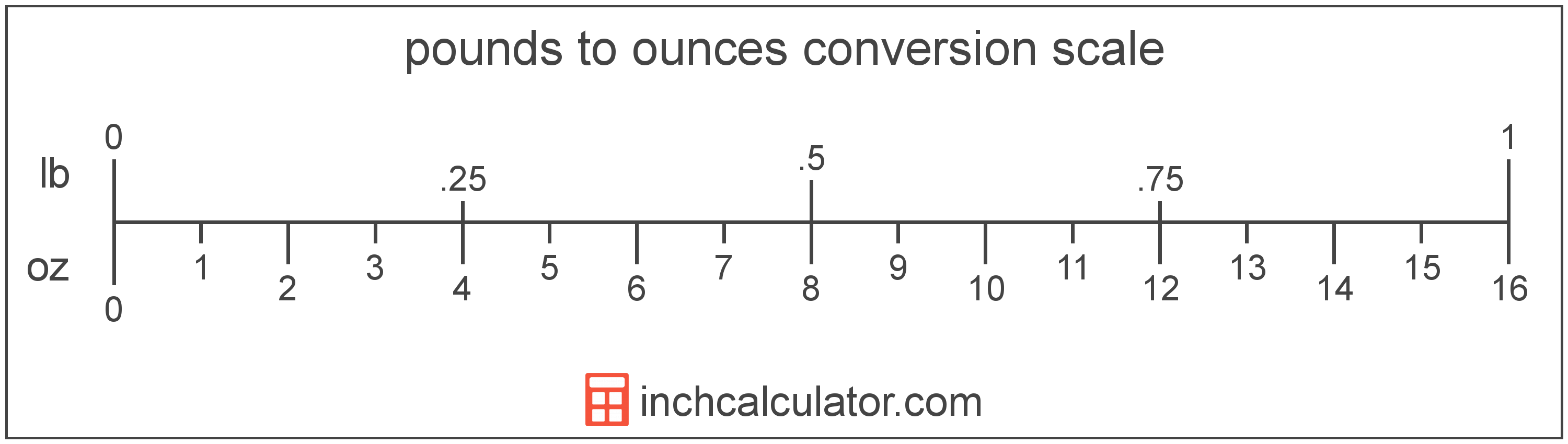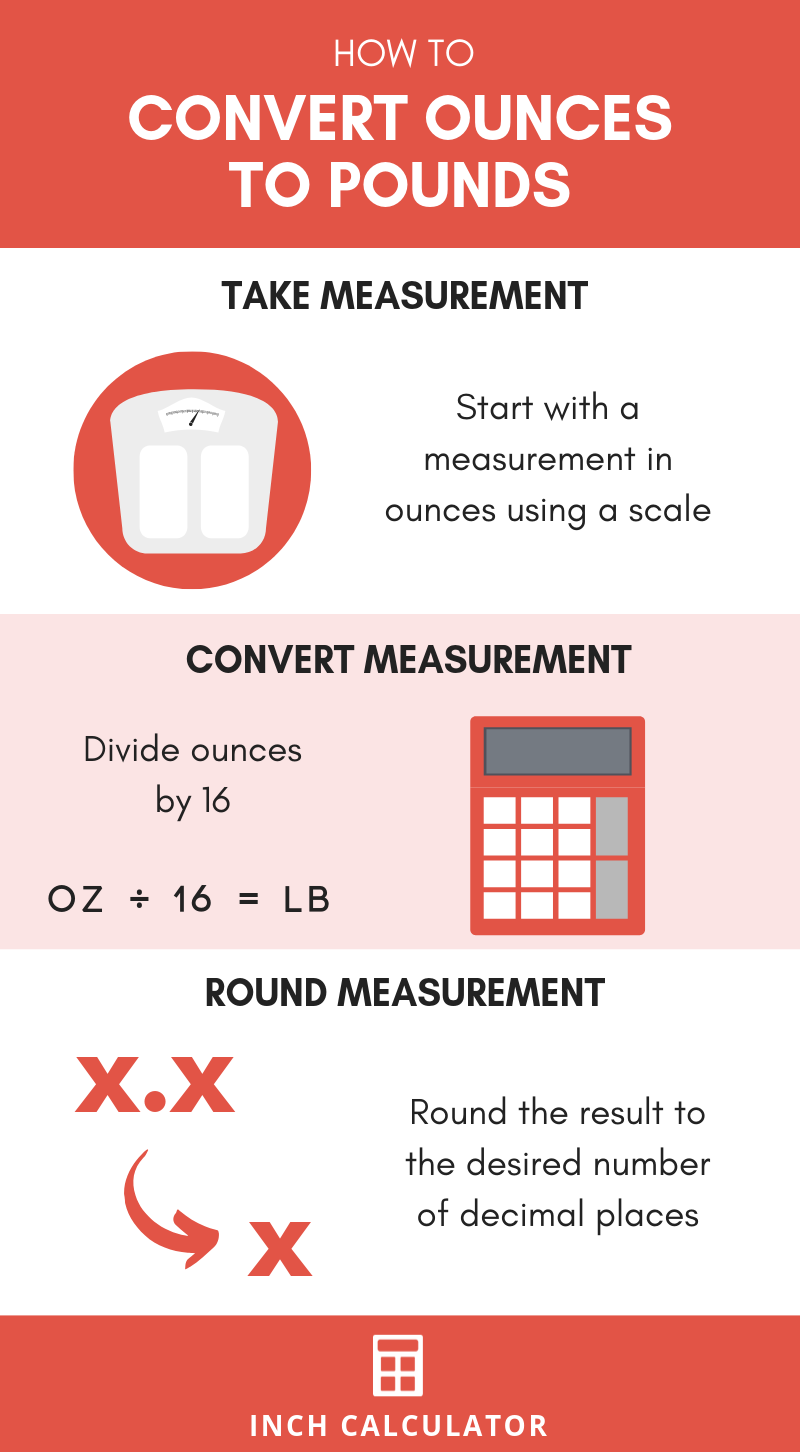# Ounces to Pounds Conversion Calculator

Enter the weight in ounces below to get the value converted to pounds.

Results in Pounds:16 oz = 1 lb

Do you want to convert pounds to ounces?

## How to Convert Ounces to Pounds

To convert an ounce measurement to a pound measurement, you can use the following ratio: 16 ounces/pound. Since one ounce is equal to 1/16 of a pound, the formula for the conversion is:

pounds = ounces ÷ 16

The weight in pounds is equal to the weight in ounces divided by 16.

For example, here's how to convert 24 ounces to pounds using the formula above.
pounds = (24 oz ÷ 16) = 1.5 lbOur oz to lbs video goes through this conversion step by step, consider watching to learn more.

### How to Convert to a Mixed Measurement

A weight measurement, in pounds, that is not a whole number is usually expressed as a measurement in pounds and ounces instead of as a decimal. For example, 5.75 lbs is often expressed as 5 lbs 12 oz.

Follow these steps to convert ounces to pounds and ounces, we'll convert 38 ounces as an example.

• Divide the total ounces by 16 to find the total pounds in decimal form
38 oz ÷ 16 = 2.375 lbs
• If the pound measurement does not have a decimal, then you're done!
• If the pound measurement does have a decimal, then we must convert the decimal remainder (the value to the right of the decimal point) to ounces. To do so, multiply the decimal remainder by 16.
0.375 × 16 = 6 oz
• Finally, combine the even pound measurement (the whole number to the left of the decimal point) and the remainder in ounces. This is the final pounds and ounces measurement.
38 oz = 2 lbs 6 oz
• A far easier way to get a mixed measurement like this is to use long division because the quotient is the whole pounds and the remainder is the ounces.## What Is an Ounce?

One ounce is a unit of mass/weight equal to 1/16 of a pound or 28.349523 grams. The common ounce should not be confused with the troy ounce, which is equal to 1/12 of a troy pound.

The ounce is a US customary and imperial unit of weight. Ounces can be abbreviated as oz; for example, 1 ounce can be written as 1 oz.

## What Is a Pound?

Pounds are a widely used unit of weight in the United States. The National Bureau of Standards approved the international definition of the pound for use in the United States in 1959 after an agreement between six nations referred to as the International Yard and Pound Agreement.

One pound is equal to 16 ounces, or 0.45359237 kilograms. In the avoirdupois or apothecaries' systems, one pound is equal to 7,000 grains.

The pound is a US customary and imperial unit of weight. A pound is sometimes also referred to as a common ounce. Pounds can be abbreviated as lb (plural lbs), and are also sometimes abbreviated as lbm or #. For example, 1 pound can be written as 1 lb, 1 lbm, or 1 #, and 2 pounds can be written as 2 lbs.

## Ounce to Pound Conversion Table

Ounce measurements converted to pounds and pounds & ounces values
Ounces Pounds Pounds & Ounces
1 oz 0.0625 lb 0 lb 1 oz
2 oz 0.125 lb 0 lb 2 oz
3 oz 0.1875 lb 0 lb 3 oz
4 oz 0.25 lb 0 lb 4 oz
5 oz 0.3125 lb 0 lb 5 oz
6 oz 0.375 lb 0 lb 6 oz
7 oz 0.4375 lb 0 lb 7 oz
8 oz 0.5 lb 0 lb 8 oz
9 oz 0.5625 lb 0 lb 9 oz
10 oz 0.625 lb 0 lb 10 oz
11 oz 0.6875 lb 0 lb 11 oz
12 oz 0.75 lb 0 lb 12 oz
13 oz 0.8125 lb 0 lb 13 oz
14 oz 0.875 lb 0 lb 14 oz
15 oz 0.9375 lb 0 lb 15 oz
16 oz 1 lb 1 lb 0 oz
17 oz 1.0625 lb 1 lb 1 oz
18 oz 1.125 lb 1 lb 2 oz
19 oz 1.1875 lb 1 lb 3 oz
20 oz 1.25 lb 1 lb 4 oz
21 oz 1.3125 lb 1 lb 5 oz
22 oz 1.375 lb 1 lb 6 oz
23 oz 1.4375 lb 1 lb 7 oz
24 oz 1.5 lb 1 lb 8 oz
25 oz 1.5625 lb 1 lb 9 oz
26 oz 1.625 lb 1 lb 10 oz
27 oz 1.6875 lb 1 lb 11 oz
28 oz 1.75 lb 1 lb 12 oz
29 oz 1.8125 lb 1 lb 13 oz
30 oz 1.875 lb 1 lb 14 oz
31 oz 1.9375 lb 1 lb 15 oz
32 oz 2 lb 2 lb 0 oz
33 oz 2.0625 lb 2 lb 1 oz
34 oz 2.125 lb 2 lb 2 oz
35 oz 2.1875 lb 2 lb 3 oz
36 oz 2.25 lb 2 lb 4 oz
37 oz 2.3125 lb 2 lb 5 oz
38 oz 2.375 lb 2 lb 6 oz
39 oz 2.4375 lb 2 lb 7 oz
40 oz 2.5 lb 2 lb 8 oz

## References

1. James Bucki, Troy Ounce vs. Ounce, The Spruce, https://www.thesprucecrafts.com/troy-ounce-vs-ounce-4588158
2. National Bureau of Standards, Refinement of Values for the Yard and Pound, U.S. Department of Commerce, July 1, 1959, https://www.nist.gov/system/files/documents/2017/05/09/frn-59-5442-1959.pdf
3. Encyclopædia Britannica, Avoirdupois weight, https://www.britannica.com/science/avoirdupois-weight